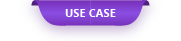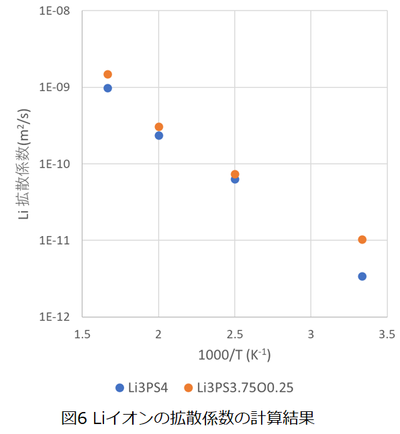In this case, we will introduce an example of analyzing the diffusion of Li ions in the solid electrolyte used in all-solid-state batteries using the neural network MD.

# Diffusion analysis of Li ions in all-solid-state battery electrolyte using neural network MD

In this case, we will introduce an example of analyzing the diffusion of Li ions in the solid electrolyte used in all-solid-state batteries using the neural network MD. When analyzing ion diffusion in a solid using first-principles calculations, the method of directly tracking the motion of ions using first-principles molecular dynamics (first-principles MD) is usually used . .. However, in order to completely reproduce the ion diffusion path using first-principles MD, it is necessary to statistically evaluate the ion distribution using the results of long-term MD calculation, so the calculation cost is very high. takes. Therefore, in this case, we evaluated the diffusion distribution of Li ions in the solid electrolyte using a neural network MD that can execute MD calculation at high speed with the same calculation accuracy as the first-principles calculation.

### 1. Training data

Here, we investigated the diffusion of Li ions into Li 3 PS 4 as a solid electrolyte and Li 3 PS 3.75 O 0.25 , which is partially oxygen-doped with Li 3 PS 4 . Figure 1 and Table 2 show the calculation model and calculation conditions used for the training data. The training data was created using the data calculated from the first principle MD using the first principle calculation code VASP. .. In addition, DeepMD  was used for the neural network MD library.

Since it is necessary to execute first-principles molecular dynamics calculations that impose a wide range of temperature and pressure conditions many times in order to create training data, it is enormous to execute the calculations sequentially in a normal cluster computer environment. Although calculation time is required, by using a cloud system, it is possible to execute all first-principles calculation degrees at the same time at the same time and perform potential learning in a short time (Fig. 2).

### 2. Potential learning result

Figure 3 shows the results of potential learning using Deep MD. With respect to the first-principles calculation results, the energy can be learned with an error of about 0.002 eV, and the force applied to the atom can be learned with an error of about 0.07 eV / Å.

Comparing the calculation time of the first-principles MD and the neural network MD, the time required for the MD1000 step is about 12000 seconds when using 36 cores, while it is 90s for the neural network MD. It was confirmed that the calculation was 100 times faster by using the neural network MD.

### 3. MD calculation conditions

The generated neural network potential data was used to evaluate the diffusivity of Li ions at Li 3 PS 4 and Li 3 PS 3.75 O 0.25 . The calculation model used in Figure 4 and the calculation conditions are shown in Table 2. LAMMPS is used for all molecular dynamics calculations. The diffusion coefficient was evaluated by the following formula using the mean square displacement of Li ions obtained by the constant energy molecular dynamics calculation (NVE).

### 4. Calculation result

An animation of the MD calculation results for Li 3 PS 4 and Li 3 PS 3.75 O 0.25 is shown on the left . At high temperature (600K) and high ion conduction state, it can be confirmed that Li ions are distributed irregularly without following the regular conduction path that jumps between the lattices, and the experimental results of Li-PS type electrolyte can be confirmed. The result is to reproduce.

Figure 5 shows the calculation results of the mean square displacement of Li ions. Comparing the results for Li 3 PS 4 and Li 3 PS 3.75 O 0.25 shows that oxygen doping increases the mean square displacement of Li ions. In addition, since the increasing tendency of the average square displacement in the low temperature state cannot be confirmed unless the simulation time is 50 ps or more, it is difficult to evaluate the ion conductivity in the low temperature state by the first-principles calculation, but in the neural network MD, ns. Since it is possible to execute a simulation for a long time, it is possible to evaluate the Li ion conductivity in a low temperature state.

Fig. 6 shows the result of evaluating the diffusion coefficient of Li ions from the calculation result of the mean square displacement obtained in Fig. 5. Comparing the results of Li 3 PS 4 and Li 3 PS 3.75 O 0.25 , it was confirmed that oxygen doping may increase the diffusivity of Li ions and improve ionic conductivity.

### 5. the next deployment

By applying this method, the following developments can be expected.

(1) Optimization of ion conductivity, dopant type, and dopant concentration in cooperation with MI

(2) Evaluation of interface resistance by neural network MD considering electrode / electrolyte interface

### 6. References

 R. Xiao, H. Li and L. Chen, “High-throughput design and optimization of fast lithium ion conductors by the combination of bond-valence method and density functional theory”, Scientfic Reports, 5, 1 (2015)

 Han Wang, Linfeng Zhang, Jiequn Han, and Weinan E. “DeePMD-kit: A deep learning package for many-body potential energy representation and molecular dynamics.” Computer Physics Communications 228 (2018): 178-184.

Original Source from: https://ctc-mi-solution.com/ニューラルネットワークmdを用いた全固体電池電解/